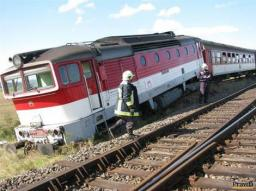# Speedboat 3189

At 12:15, a train speeding 55km/h left the station. Twelve minutes later, an 80km/h speedboat set off behind the train. What time does the express train catch up? How far from the station?

Result

t = 12:53 hh:mms =  35.2 km

### Step-by-step explanation:

s=55·(t-(12+15/60))
s = 80·(t-(12+(15+12)/60))

60s-3300t = -40425
60s-4800t = -59760

Row 2 - Row 1 → Row 2
60s-3300t = -40425
-1500t = -19335

t = -19335/-1500 = 12.89
s = -40425+3300t/60 = -40425+3300 · 12.89/60 = 35.2

s = 176/5 = 35.2
t = 1289/100 = 12.89

Our linear equations calculator calculates it.Did you find an error or inaccuracy? Feel free to write us. Thank you!

Tips for related online calculators
Do you want to convert length units?
Do you want to convert velocity (speed) units?
Do you want to convert time units like minutes to seconds?